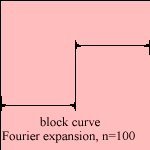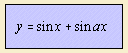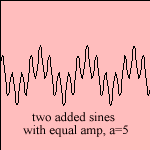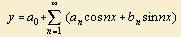# sine summation

## trigonometricEach periodic function f that is adjusted, can be written as a infinite sum of sine and cosine terms. It was Fourier (1768-1830) who was the first to realize this, so that this infinite sum is called a Fourier series 1). This vanished the difference between function and curve: each function has a curve, and for each curve there is a function (its Fourier expansion).
For odd f, the expansion contains only sine terms (sine series), for even f only cosine terms (cosine series).
The function f need not to be periodic. In that case we take into account the interval [a, b], to be seen as the period of the curve.

While a function has convergence in each point, differences are possible between the graph of a function and its Fourier expansion. According to the effect of Gibbs, at a discontinuity the amplitude is too large, for the Fourier expansion. This is illustrated in a picture for a block curve and its Fourier expansion after 100 terms.An easy example of adding sines is when there are two sines with same amplitude. This is the situation of two harmonic oscillations with the same power. The patterns of the sum are periodic when the ratio of the two wave lengths (a) is rational.
A musical tone is a combination of a fundamental tone (with frequency f) with its overtones (with frequencies n*f). The proportion of the amplitudes makes the 'timbre' of the tone.

notes

1) The Fourier expansion for a function f with period 2 π:According to Fourier theory the terms a and b can be expressed in an integral in f and cos(nx) and sin(nx), respectively.